# Overview of Linear Equations: Tutoring Solution Chapter Exam

Exam Instructions:

Choose your answers to the questions and click 'Next' to see the next set of questions. You can skip questions if you would like and come back to them later with the yellow "Go To First Skipped Question" button. When you have completed the practice exam, a green submit button will appear. Click it to see your results. Good luck!

Answered 0 of 30

### Page 1

#### Question 4 4. What is the solution to the system of equations shown in the graph below?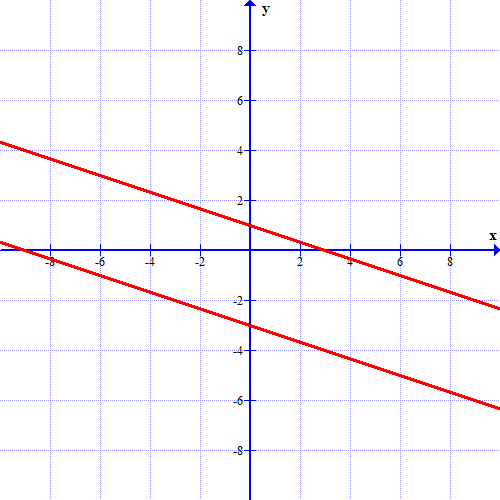### Page 2

#### Question 9 9. What is the slope-intercept form equation of the graph below?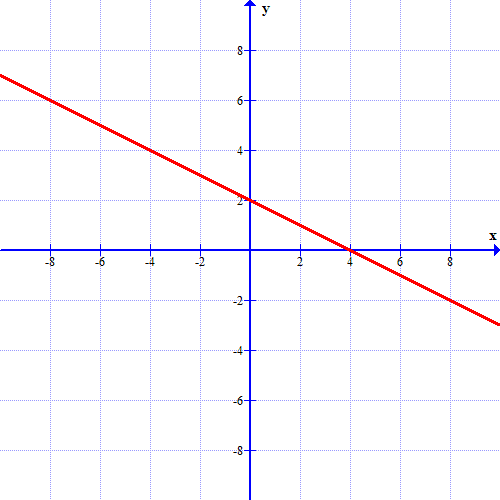### Page 3

#### Question 12 12. Look at the graph. What is the solution to the system of equations?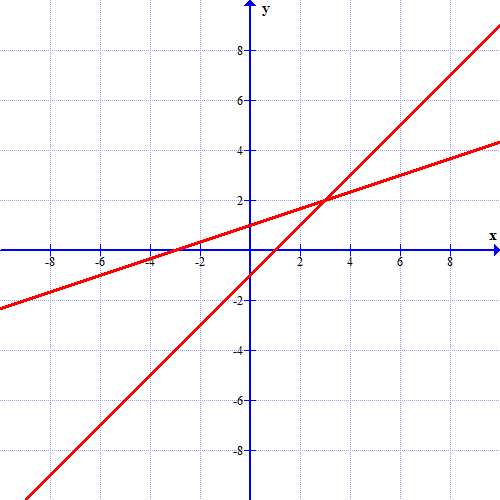#### Question 15 15. Which of these equations represents a line that is perpendicular to the line in the graph below?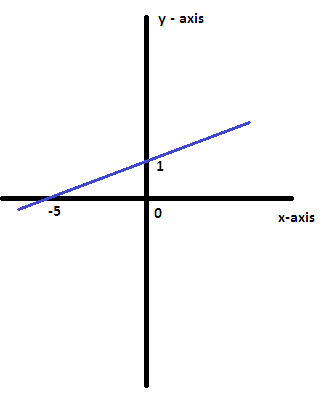### Page 4

#### Question 16 16. If the graph below has the equation Ax + By = -4, what must be true about A and B?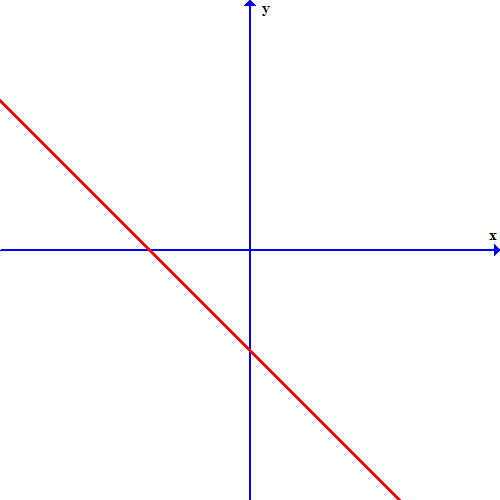### Page 6

#### Question 29 29. The equation for the following graph could be _____.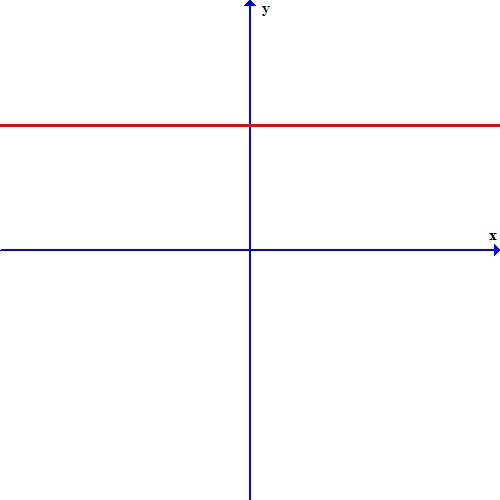#### Overview of Linear Equations: Tutoring Solution Chapter Exam Instructions

Choose your answers to the questions and click 'Next' to see the next set of questions. You can skip questions if you would like and come back to them later with the yellow "Go To First Skipped Question" button. When you have completed the practice exam, a green submit button will appear. Click it to see your results. Good luck!

Support